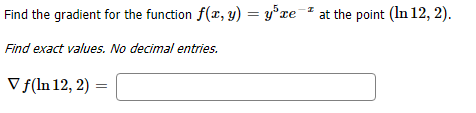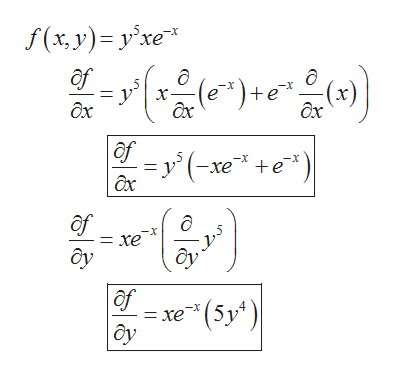# at the point (In 12,2)Find the gradient for the function f(a, y) = y'xe #Find exact values. No decimal entriesVf(In 12, 2)

Question
1 viewshelp_outlineImage Transcriptioncloseat the point (In 12,2) Find the gradient for the function f(a, y) = y'xe # Find exact values. No decimal entries Vf(In 12, 2) fullscreen
check_circle

Step 1

The given function whose gradient is to be calculated at a given point is:

Step 2

If w=f(x, y) then,

Step 3

Now, find the partial derivative of fun...help_outlineImage Transcriptionclosef(x, y) y'xe f e)+e x ax ax дх af y'(xee дх -x = xe ôy af xe (5y) fullscreen

### Want to see the full answer?

See Solution

#### Want to see this answer and more?

Solutions are written by subject experts who are available 24/7. Questions are typically answered within 1 hour.*

See Solution
*Response times may vary by subject and question.
Tagged in

### Calculus